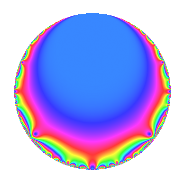# Properties

 Label 59.1.bLevel 59 Weight 1 Character orbit b Rep. character $$\chi_{59}(58,\cdot)$$ Character field $$\Q$$ Dimension 1 Newforms 1 Sturm bound 5 Trace bound 0

# Related objects

## Defining parameters

 Level: $$N$$ = $$59$$ Weight: $$k$$ = $$1$$ Character orbit: $$[\chi]$$ = 59.b (of order $$2$$ and degree $$1$$) Character conductor: $$\operatorname{cond}(\chi)$$ = $$59$$ Character field: $$\Q$$ Newforms: $$1$$ Sturm bound: $$5$$ Trace bound: $$0$$

## Dimensions

The following table gives the dimensions of various subspaces of $$M_{1}(59, [\chi])$$.

Total New Old
Modular forms 2 2 0
Cusp forms 1 1 0
Eisenstein series 1 1 0

The following table gives the dimensions of subspaces with specified projective image type.

$$D_n$$ $$A_4$$ $$S_4$$ $$A_5$$
Dimension 1 0 0 0

## Trace form

 $$q$$ $$\mathstrut -\mathstrut q^{3}$$ $$\mathstrut +\mathstrut q^{4}$$ $$\mathstrut -\mathstrut q^{5}$$ $$\mathstrut -\mathstrut q^{7}$$ $$\mathstrut +\mathstrut O(q^{10})$$ $$q$$ $$\mathstrut -\mathstrut q^{3}$$ $$\mathstrut +\mathstrut q^{4}$$ $$\mathstrut -\mathstrut q^{5}$$ $$\mathstrut -\mathstrut q^{7}$$ $$\mathstrut -\mathstrut q^{12}$$ $$\mathstrut +\mathstrut q^{15}$$ $$\mathstrut +\mathstrut q^{16}$$ $$\mathstrut +\mathstrut 2q^{17}$$ $$\mathstrut -\mathstrut q^{19}$$ $$\mathstrut -\mathstrut q^{20}$$ $$\mathstrut +\mathstrut q^{21}$$ $$\mathstrut +\mathstrut q^{27}$$ $$\mathstrut -\mathstrut q^{28}$$ $$\mathstrut -\mathstrut q^{29}$$ $$\mathstrut +\mathstrut q^{35}$$ $$\mathstrut -\mathstrut q^{41}$$ $$\mathstrut -\mathstrut q^{48}$$ $$\mathstrut -\mathstrut 2q^{51}$$ $$\mathstrut -\mathstrut q^{53}$$ $$\mathstrut +\mathstrut q^{57}$$ $$\mathstrut +\mathstrut q^{59}$$ $$\mathstrut +\mathstrut q^{60}$$ $$\mathstrut +\mathstrut q^{64}$$ $$\mathstrut +\mathstrut 2q^{68}$$ $$\mathstrut +\mathstrut 2q^{71}$$ $$\mathstrut -\mathstrut q^{76}$$ $$\mathstrut -\mathstrut q^{79}$$ $$\mathstrut -\mathstrut q^{80}$$ $$\mathstrut -\mathstrut q^{81}$$ $$\mathstrut +\mathstrut q^{84}$$ $$\mathstrut -\mathstrut 2q^{85}$$ $$\mathstrut +\mathstrut q^{87}$$ $$\mathstrut +\mathstrut q^{95}$$ $$\mathstrut +\mathstrut O(q^{100})$$

## Decomposition of $$S_{1}^{\mathrm{new}}(59, [\chi])$$ into irreducible Hecke orbits

Label Dim. $$A$$ Field Image CM RM Traces $q$-expansion
$$a_2$$ $$a_3$$ $$a_5$$ $$a_7$$
59.1.b.a $$1$$ $$0.029$$ $$\Q$$ $$D_{3}$$ $$\Q(\sqrt{-59})$$ None $$0$$ $$-1$$ $$-1$$ $$-1$$ $$q-q^{3}+q^{4}-q^{5}-q^{7}-q^{12}+q^{15}+\cdots$$